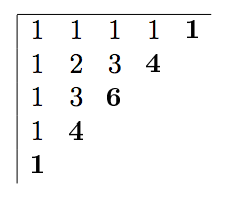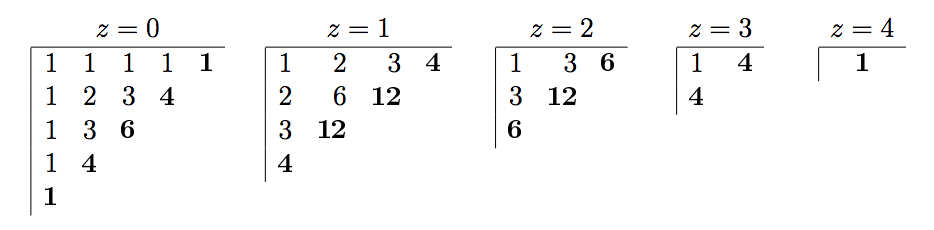# 3224. 帕斯卡金字塔

$D$ 维的帕斯卡金字塔，是一个具有如下定义的函数：$x=1, y=2, z=1$ 为例，$(1,2,1)$ 的值是 $(0,2,1)$$(1,1,1)$$(1,2,0)$ 的和，也就是 $6+3+3=12$。金字塔的底层是满足 $x+y+z=4$ 的部分，即加粗的部分。

### 样例

Input
2 5

Output
1
4
6

Input
3 5

Output
1
4
6
12


4 人解决，4 人已尝试。

5 份提交通过，共有 15 份提交。

9.6 EMB 奖励。